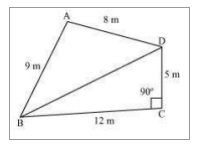# NCERT Solutions Class 9 Mathematics Solutions for Heron’s Formula - Exercise 12.2 in Chapter 12 - Heron’s Formula

Question 1 Heron’s Formula - Exercise 12.2

A park, in the shape of a quadrilateral ABCD, has ∠C = 90°, AB = 9 m, BC = 12 m, CD = 5 m

and AD = 8 m. How much area does it occupy?

First, construct a quadrilateral ABCD and join BD.

We know that

∠C = 90°, AB = 9 m, BC = 12 m, CD = 5 m and AD = 8 m

The diagram is:Now, apply Pythagoras theorem in ΔBCD

\begin{aligned} &B D^{2}=B C^{2}+C D^{2}\\ &\Rightarrow B D^{2}=12^{2}+5^{2}\\ &\Rightarrow B D^{2}=169\\ &\Rightarrow B D=13 \mathrm{m}\\ &\text { Now, the area of } \Delta B C D=(1 / 2 \times 12 \times 5)=30 \mathrm{m}^{2} \end{aligned}

The semi perimeter of ΔABD

(s) = (perimeter/2)

= (8 + 9 + 13)/2 m

= 30/2 m = 15 m

Using Heron's formula,

Area of ΔABD

\begin{array}{l} \sqrt{s(s-a)(s-b)(s-c)} \\ =\sqrt{15(15-13)(15-9)(15-8)} m^{2} \\ =\sqrt{15 \times 2 \times 6 \times 7 m^{2}} \\ =6 v 35 \mathrm{m}^{2}=35.5 \mathrm{m}^{2} \text { (approximately) } \end{array}

=30 \mathrm{m}^{2}+35.5 \mathrm{m}^{2}=65.5 \mathrm{m}^{2}

Video transcript
"hello students welcome to lido q a video session i am seth your math tutor and question for today is a part in a shape of quadrilateral abcd has angle c is equal to 90 degree a b is equal to 9 meter dc is equal to 12 meter cd is equal to 5 meter and 80 is equal to 8 meter how much area does it occupy so the park layout is shown here below abcd is the layout we know from the question that angle c is 90 so angle c is equal to 90. a b is equal to 9 meter bc is equal to 12 meter cd is equal to 5 meter and 80 equals to 8 meter now you have to construct the quadrilateral as shown here that is body little abcd in this quadrilateral you have to join the diagonal bd so here we have joined the bd now apply pythagoras theorem in triangle bcd triangle bcd is a right angle triangle because it is given that angle c is 90 degree so as per pythagoras theorem you can say that bd square is equal to bc square plus cd square we need to find bd so bd square will be equal to 12 square plus y square and which is equal to 169 root of 169 is bd hence bd is equal to 13 meter now area of triangle bcd can be given by half into base into height base here is pc and height is dc so it is half into 12 into phi so we will continue here that is area of triangle bcd that is a is equal to half into 12 into 5 that is 30 meter square now semi perimeter of triangle abd will be semi perimeter s of triangle abd s is equal to perimeter upon 2 that is semi perimeter 8 plus 9 plus 13 upon 2 and that is 30 upon 2 so s is equal to 15 meter now once you have found the semi perimeter you can use the herons formula to find the area so as per the herons formula area of triangle area of triangle abd can be given by under root of as s minus a s minus b s minus c so 15 under root of 15 15 minus 8 15 minus 9 and 15 minus 13 so that is under root of 15 into 2 into 6 into 7 and that is meter square and that comes out to be 6 root 35 approx will be so your area of triangle abd will be equal to 6 root 35 and that is equal to area will be 35.5 meter square approx now what you need to do is add both the triangles area so area of quadrilateral a b c b that is the area of the park will be equal to area of triangle abd plus area of triangle bcd previously we have found the area of triangle bcd to be 30 meter square and area of triangle abd just now we have found as 35.5 meter square so abd plus bcd will be equal to 35.5 plus 30 and hence this will give you area of the part which is equal to 65.5 meter square so that is our required area if you have any query you can drop it in our comment section and subscribe to lido for more such q a thank you for watching "
Related Questions

Lido

Courses

Teachers

Book a Demo with us

Syllabus

Maths
CBSE
Maths
ICSE
Science
CBSE

Science
ICSE
English
CBSE
English
ICSE
Coding

Terms & Policies

Selina Question Bank

Maths
Physics
Biology

Allied Question Bank

Chemistry
Connect with us on social media!